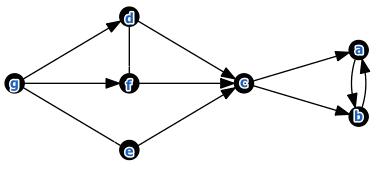# ML Wiki

## Complete Pre-Order

This is a preference structure for Modeling Preferences in MCDA

Also called the traditional representation of preferences

$\forall a,b \in A:$

• $a \ P \ b \iff g(a) > g(b)$ (The complete order: $R$ relation)
• $a \ I \ b \iff g(a) = g(b)$ (The complete pre-oder: $I$ relation)

$g$ is some global aggregation function:

This way we cannot model incomparability ($>$ can always compare things)

• $J$ is always empty - everything is comparable
• $P$ is transitive
• $I$ also becomes transitive

### Example 1

Suppose there are three sport teams: $a$, $b$, and $c$.

• If $a$ beats $b$, $a$ receives 3 points and the loser receives 0 points
• If they draw, both receives 1 point.
• The three teams will play with each other and at the end they will have total points.
• If all total scores are different, there will be a complete order
• If there is a tie, the order will be a complete preorder

### Example 2

Expected gains of different actions:

$a$ $b$ $c$ $d$ $e$ $f$ $g$
100 100 120 130 130 130 131